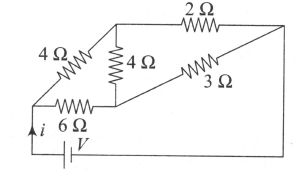For the network shown in the figure, the value of the current i is

# For the network shown in the figure, the value of the current i is1. A

$\frac{9V}{35}$

2. B

$\frac{5V}{18}$

3. C

$\frac{5V}{9}$

4. D

$\frac{18V}{5}$

Register to Get Free Mock Test and Study Material

+91

Verify OTP Code (required)

### Solution:

The circuit given resembles the balanced Wheatstone bridge as $\frac{4}{6}\text{\hspace{0.17em}}=\frac{2}{3}$

Thus, middle anm containing $4\text{\hspace{0.17em}\hspace{0.17em}}\Omega$ resistance will be ineffective and no current flows through it.
The equivalent circuit is shown as below:

Net resistance of AB and BC,

${R}^{\text{'}}=4+2=6\text{\hspace{0.17em}\hspace{0.17em}}\Omega$

Net resistance of AD and DC,

${R}^{\text{'}\text{'}}=6+3=9\text{\hspace{0.17em}\hspace{0.17em}}\Omega$

Thus, parallel combination of R' and R" gives

$\mathrm{R}=\text{\hspace{0.17em}\hspace{0.17em}}\frac{{\mathrm{R}}^{\text{'}}\text{\hspace{0.17em}}×\text{\hspace{0.17em}}{\mathrm{R}}^{\text{'}\text{'}}\text{\hspace{0.17em}}}{{\mathrm{R}}^{\text{'}}\text{\hspace{0.17em}}+\text{\hspace{0.17em}}{\mathrm{R}}^{\text{'}\text{'}}}\text{\hspace{0.17em}\hspace{0.17em}}=\text{\hspace{0.17em}\hspace{0.17em}}\frac{6\text{\hspace{0.17em}\hspace{0.17em}}×\text{\hspace{0.17em}\hspace{0.17em}}9}{6+9}\text{\hspace{0.17em}\hspace{0.17em}}=\text{\hspace{0.17em}\hspace{0.17em}}\frac{54}{15}\text{\hspace{0.17em}\hspace{0.17em}}=\text{\hspace{0.17em}\hspace{0.17em}}\frac{18}{5}\text{\hspace{0.17em}\hspace{0.17em}}\mathrm{\Omega }$

Hence, current  $\mathrm{i}=\frac{\mathrm{V}}{\mathrm{R}}=\frac{\mathrm{V}}{18/5}\text{\hspace{0.17em}\hspace{0.17em}}=\frac{5\mathrm{V}}{18}$Register to Get Free Mock Test and Study Material

+91

Verify OTP Code (required)